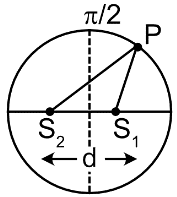Condition for Interference
Question

# Two radio frequency point sources ${S}_{1}$  and ${S}_{2}$ , separated by distance 2.5 m are emitting in phase waves of wavelength 1 m. A detector moves in a large circular path around the two sources in a plane containing them. The number of maxima that will be detected by it over the complete circular path, areModerate
Solution

## At point A, the path difference is 2.5$\lambda$ and at B, path difference is zero. While moving from A to B , path difference changes from 2.5 to zero, making two maxima corresponding to 2 $\lambda$ and $\lambda$  (excluding). B is common for both upper quarter circles hence there are five maxima in one half circle, making 10 in total circular movement.

Get Instant Solutions